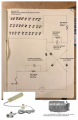# Help Rewiring a Machine Light

Thread Starter

#### edentan

Joined Jan 9, 2021
1
Dear All About Circuits,

Recently I bought a lamp for my industrial sewing machine which runs on AC Mains power (Rated at either 110V or 220V), for the sake of having the lamp power on when I power on the Sewing machine (and for a bit of fun) I wanted to reconfigure it so that it connected into the outlet on the back of the sewing machine (bottom right picture). I have no education in electronics so through a lot of googling I have got to this point, but am now at a loss.

Below is a circuit diagram of how the light works, I have become stuck trying to measure the forward voltage across each LED light, all my multimeter shows me is the number 1. Instead I have tried to provide as much information as possible, such as measurements taken from across the 30x LEDs says that they are drawing 28V and 0.04A, meaning that they have a resistance of 700 Ohms I think.

I would really appreciate someone telling me if it is possible for me to reconfigure this light so that it can connect it to the 5VDC, 1W, <100ma outlet on the back of my sewing machine and if so any ideas how. Do I need a DC-DC voltage booster? If i did would it even be possible? Argh!!

p.s. my idea if i can't is to wire the lamp and sewing machine into a junction box and then use a relay plugged into the outlet which will power on the LEDs which I'm pretty sure will work!Thank you for any help what so ever!

#### LesJones

Joined Jan 8, 2017
3,168
From my calculations (Based on the reactance of the 680 nF capacitor.) if supplied from 240 volt 50 Hz mains the light will take about 0.7 watts. (If it was run on 120 volt mains it would be much less so it will not be very bright even on 240 volts. I don't think your current reading is correct and you did not say if that was the total current for the three chains or 1 of the three chains. I suspect that you measured the current with the LEDs shorted out by your meter set to a current range. My calculations give a current of about 8mA through each chain. I am assuming that the lamp uses white LEDs so there forward voltage will be about 3.0 to 3.3 volts. So to run them from 5 volts (Based on 3.3 volts forward voltage you would require a resistor of (5.0 - 3.3)/0.008 = 212.5 ohms in series with each LED. 220 ohms is the nearest standard value. You would have to totally rewire the LEDs on the printed circuit board so that you had the 30 LEDs each with it's own 220 ohm current limiting connected in parallel. This would not be a simple task. It would also exceed the current rating of the 5 volt socket. If you used 470 ohm resistors it would be within the 5 volt socket rating but the light would be dimmer. I think it would be much simpler to use a relay with the coil powered from the 5 volt socket on the machine to switch the light on.

Les.

Similar threads